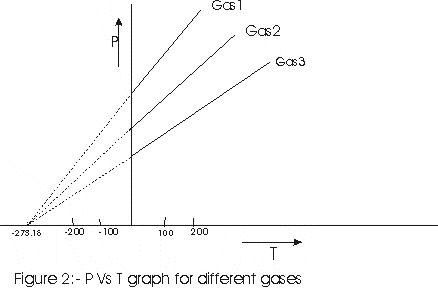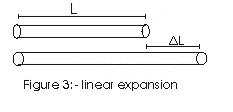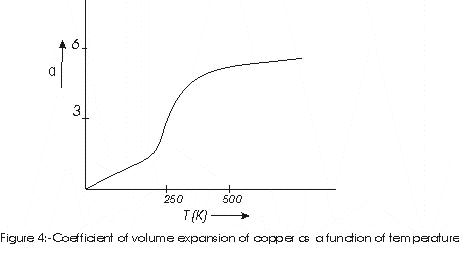# Thermal Properties of Matter

## 4. Ideal Gas Equation and Absolute Temperature

### 4.1 Ideal Gas Equation

Pressure of all the gases changes with the temperature in a similar fashion for low temperature. Also many properties of gases are common at low pressure. The pressure, volume and temperature in kelvin of such gases obey the equation \begin{equation} PV=nRT \tag{1} \end{equation} where $n$ is amount of gas in number of moles and is given as , $$n = \left( {\frac{{{\textrm{Total number of molecules in given mass of gas}}}}{{{\textrm{Avagadro Number}}{{\textrm({N}}_A})}}} \right)$$ where, $N_{A}=6.023\times 10_{23}$ $R$ is universal gas constant and its value is $R=8.316$ J/mole-K. Equation (1) is known ideal gas equation and a gas obeying this equation is known ideal gas equation.

### 4.2 Absolute Temperature

Suppose we calibrate two thermometers, for example, the liquid in a tube and a resistance thermometer. Here they agree at $0^0C$ and $100^0C$, but they may not agree exactly at intermediate temperatures. Any temperature scale defined in this way depends on the specific properties of the material used. We want to define a temperature scale which does not depend on the properties of a particular material. Here we will discuss a thermometer that comes close to ideal. That thermometer is a Gas thermometer. A thermometer that uses a gas gives the same reading regardless of the gas used.
Principle of a gas thermometer :- The pressure of a gas at constant volume increases with temperature.
In gas thermometer , a quantity of gas is placed in a constant volume container. Then we measure its pressure. To calibrate a constant volume gas thermometer, we measure pressure at $0^C$ and $100^C$. We now plot these points on a graph and draw straight line between these two points. From this graph we can read the temperature corresponding to other pressure. Figure below show the result of three such experiments with different gases.It is found that when graph were extrapolated for temperature below $0^{\circ}C$, pressure comes out to be zero at $-273^{\circ}C$ and this is the lowest temperature. Lord kelvin suggested that instead of $0^{\circ}C$ which is the melting point of ice,$-273^{\circ}C$ should be regarded as the zero of the temperature scale. Such a scale of temperature is absolute scale of temperature and $-273.15^{\circ} C$ as absolute zero of this new scale and is denoted as $0K$ and steam point in this scale correspond to $373.15 K$. From various experiments it turns out that this temperature is same for many different gases, at least in the limit of very low gas density. In gas thermometer different gases are used for measuring high temperatures and temperature reading are found to be independent of the nature of the gas used.
Gas thermometer can also define Celsius scale. If $P_{0}$ is pressure of gas at ice point and $P_{100}$ is pressure of gas at steam point then temperature T corresponding to a pressure P of gas is defined by, $$T=\frac{(P-P_{0})100}{(P_{100}-P_{0})}$$

## 5. Thermal Expansion

Most of the solid material expands when heated. Increase in dimension of a body due to increase in its temperature is called thermal expansion. For small change in temperature $\Delta T$ of a rod of length $L$, the fractional change in length $\frac{\Delta L}{L}$ is directly proportional to $\Delta T$(Fig 3)\begin{equation} \frac{\Delta L}{L}=\alpha_{L}\Delta T \tag{2} \end{equation} or, \begin{equation} \Delta L=\alpha_{L}L\Delta T \tag{3} \end{equation} Constant $\alpha_L$ characterizes the thermal expansion properties of a particular material and it is known as coefficient of linear expansion. For materials having no preferential direction, every linear dimension changes according to equation (3) and $L$ could equally well represent the thickness of the rod, side length of the square sheet etc. Normally metals expand more and have high value of $\alpha$. Again consider the initial surface area $A$ of any surface and $A'$ is the area of the solid when the temperature of the body changes by $\Delta T$ then increase in surface area is given by \begin{equation} \Delta A =\alpha_{A}A\Delta T \tag{4} \end{equation} where $\alpha_{A}$ is the coefficient of area expansion.
Similarly, we can define coefficient of volume expansion as fractional change in volume $\frac{\Delta V}{V}$ of a substance for a temperature change $\Delta T$ as \begin{equation} \alpha_{V}=\frac{\Delta V}{V\Delta T} \tag{5} \end{equation} $K^{-1}$ is the unit of these coefficients of expansions. These three coefficient are not strictly constant for a substance and there value is depends on temperature range in which they are being measured. As an example, figure below shows that coefficient of volume expansion increase with temperature and takes a constant value above $500K$### Relation between volume and linear coefficient of expansion for solid material:

Consider a solid parallelepiped with dimension $L_1$,$L_2$ and $L_3$ then its volume is $$V= L_{1}L_{2}L_{3}$$ When temperature increase by a amount $\Delta T$ then each linear dimension changes and then new volume is \begin{align*} V+\Delta V &=L_{1}L_{2}L_{3}(1+\alpha_{L}\Delta T)^{3}\\ &= V(1+\alpha_{L}\Delta T)^{3}\\ &= V(1+3\alpha_{L}\Delta T+3\alpha_{L}2(\Delta T)^{2}+\alpha_{L}3(\Delta T)^{3}) \end{align*} if $\Delta T$ is small then higher order of $\Delta T$ can be neglected. Thus we find $$V+\Delta V=V(1+3\alpha_{L}\Delta T)$$ or, $$\Delta V=3\alpha_{L}V_{0}\Delta T$$ Comparing this with equation (5) we find $$\alpha_{V}=3\alpha_{L}$$

link to this page by copying the following text

Note to our visitors :-

Thanks for visiting our website.
DISCLOSURE: THIS PAGE MAY CONTAIN AFFILIATE LINKS, MEANING I GET A COMMISSION IF YOU DECIDE TO MAKE A PURCHASE THROUGH MY LINKS, AT NO COST TO YOU. PLEASE READ MY DISCLOSURE FOR MORE INFO.